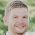## Friday, May 12, 2017

### 1D Clustering with KDE

Kernel Density Estimation (KDE) is a useful technique for clustering one-dimensional data. For example, I recently implemented an interface for clustered parallel coordinates, in which I needed to cluster about 600k variables at the click of a button:

Of course, KDE requires a lot of expensive operations. Making the above happen in a few seconds required a few efficiency tricks at the cost of perfectly accurate clusters. So, I put together this mini-tutorial to explain my approach.

#### What is Kernel Density Estimation?

KDE helps identify the density of a distribution of data. That is, it can be useful in finding where a lot of data is grouped together and where it isn't. Naturally, it can be used for 1D clustering by creating clusters about the points of highest density (local maxima), separated by the points of lowest density (local minima). There are many great online tutorials about KDE, and I recommend familiarizing yourself before moving on.

1D clustering with KDE can be done in roughly 4 steps:
1. Normalize data (0 to 1) and sort
2. Compute densities
3. Find local maxima
4. Find minima and cluster
For all of my examples I'll be using Matlab with the final script linked at the bottom. Because it's Matlab it will be slow. However, the methods translate well to other languages.

#### 1.) Normalize data and sort

With standard KDE you don't need to sort, because density is calculated from every other point in your dataset. Since the following section makes use of a limited neighborhood, sorting is necessary.

#### 2) Compute densities

This is the only step that requires a little bit of work and some knowledge of KDE. It's also where we can start taking shortcuts for faster clustering.

The general idea is for a given point along the data, we compare the distance of that point to its neighbors. Neighbors that are really close add more to the density and neighbors that are far away add much less. How much they add is dependent on the choice of a smoothing kernel with radius h. A good choice for h is the Silverman's rule of thumb: h=std(x)*(4/3/n)^(1/5). For example, the density at point i, data x, number of elements n, and often-used Gaussian/normal kernel would be:

sum = 0;
for j = 1:n
v = ( x(i) - x(j) )/h;

sum = sum + exp(-0.5*v*v) / sqrt(2*pi);
end
density = sum/(n*h);

For full KDE, that's an unseemly n^2 checks. To reduce the workload, we can make two simplifications:
1. Compute density in bins instead of every point
2. Check only nearby neighbors that have a greater impact on density
For a small dataset this is a little unnecessary. But consider 600k with 10 bins and checking 100 neighbors: you've reduced the loop from 360 billion to 1000. The choice of number of bins and neighbors is dependent on application. Less bins gives you less clusters. Less neighbors will dramatically improve run time but may give you noisier results, especially when an ignored neighbor would add a not-so-insignificant amount to the density. Sometimes is sufficient to add a round of smoothing, where the density of a bin is recomputed as the average of itself and left and right bins. The following code snippet applies these simplifications:

h = std(x) * (4/(3*n))^(1/5);
nn = 10; % number of neighbors to check on either side
nb = 10; % number of bins
bh = 1/10; % bin step size
bins = zeros(nb,1); % nb bins for computing density
for i = 1:n

bin = ceil(x(i)/bh); % bin index
sum = 0;
for j = max(1,i-nn):min(n,i+nn)
v = (x(i)-x(j))/h;

sum = sum + exp( -0.5*v*v ) / sqrt(2*pi);
end
dens = sum/(2*nn*h);
bins(bin) = bins(bin) + dens;
end
for i = 2:nb-1 % Smoothing
bins(i) = (bins(i-1)+bins(i)+bins(i+1))/3;
end

Of course, the bins aren't exactly centered at the points of highest density. For a lot of applications this is perfectly fine. Plotting the resulting density and data gives us:

#### 3) Find local maxima

Local maxima are the peaks of the curves in the above plot. They can easily be discovered by checking the bins on either side. If it's the largest, it's a local maximum! If your curve is jaggy with too many maxima, you'll need to increase the number of neighbors sampled when computing density. Play with the tunable variables until you have something that looks nice:

#### 4) Find local minima

The minima are simply the bins of lowest density between two maximums:

Local minima define where the clusters split. In the above example, we can see the data is split into three clusters.

And that's it. The script I used to create the plots above can be found right here.

1.Is there any publication paper that I can refer KDE for 1-D clustering?

2.I need the reference to support my publication

3.I see. Unfortunately I don't have a reference. KDE is a pretty standard method and I just used some hacks to make it work fast. I would actually recommend a different method for 1D clustering (finding extrema) that does have a citation: https://people.mpi-inf.mpg.de/~weinkauf/notes/persistence1d.html

4.Thanks for the recommendation